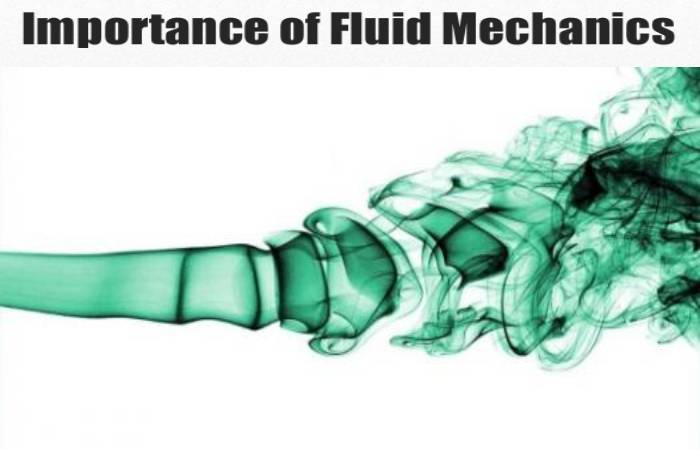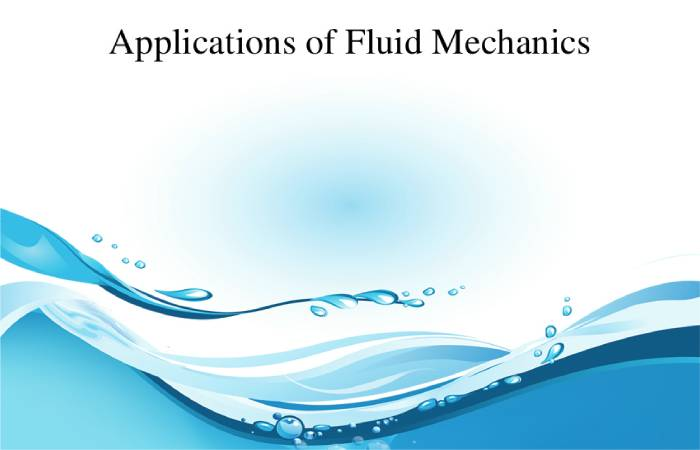# What is Fluid Mechanics? – Types, Equations Governing, and More

## What is Fluid Mechanics?

Fluid mechanics, as its name implies, is an area of ​​mechanics aimed at studying fluids. To achieve his goal, he uses the principles of classical mechanics.

Fluid mechanics is linked directly to engineering, from mechanical engineering, to hydraulic, through construction.

## Types of Fluid Mechanics

The study of fluid mechanics considers two types of fluids:

### 1. Fluid at Rest

• They exert a force on the containers’ walls containing This force is known as hydrostatic pressure.

### 2. Fluid in Motion

• They are fluids that are not static. An example of this is air currents, water in a river or coming out through a pipe.
• The study of moving liquids is known as hydrodynamics while moving gases is known as aerodynamics.
• Fluid mechanics is the branch of physics responsible for studying fluids and the forces that cause them.
• Fluid mechanics also studies the interactions between the fluid and the surrounding environment.
• It is based on various laws, among which we can highlight the first and second laws of thermodynamics and the rule of conservation of mass and momentum.
• The study of fluid mechanics starts from hypotheses, which allow the development of concepts.

### 3. Continuous Medium Hypothesis

• It is the fundamental basis of all fluid mechanics and allows the development of all the concepts.
• It maintains that the fluid is continuous throughout it occupies, ignoring its molecular structure and discontinuities.
• This hypothesis considers that the properties of the fluid are continuous (density, temperature, etc.).

### 4. Fluid Particle

• It is one of the most relevant fluid mechanics concepts, closely related to the continuous medium’s hypothesis.
• We can define a fluid particle as the elemental mass of a fluid that is at a point in space at an instant of time.
• This mass must be large enough to be integrated by many molecules and small sufficient to consider no variations in the fluid’s macroscopic properties.
• The fluid particle is in motion with the same macroscopic speed as the fluid since it is made up of the same molecules.
• It should note that a specific point in space at different time instants will be made up of varying fluid particles.

### 5. Descriptions of Fluid Motion

• We can find a couple of different views during fluid motion study: Lagrangian description and Eulerian description.

#### Description Langragiana

• It is based on following each of the fluid particles in their movement to find the functions.
• And also, it allows us to describe the position of said particles and each fluid particle’s properties at each instant of time of the study.

#### Description Eulerian

• It consists of assigning each point in space, at each instant of study time, a unique value for the fluid properties without considering that the fluid particle occupies that differential volume.
• As we can see, it is not linked to the fluid particle but the spatial points occupied by the fluid instantly. This description is the most used to deduce and obtain the general equations of fluid mechanics.

## Equations Governing Fluid Mechanics

The study of  ​​the science area, the use of equations, requires describing the fluid’s movement in this case. To derive these equations, one must apply the principle of conservation of energy and thermodynamics laws to a liquid volume.

Likewise, these equations must be generalized. Thus, the Gaussian Theorem and the Reynolds Transport Theorem are used to obtain much more manageable and valuable equations for the Eulerian formulation. There are mainly three basic equations for the study of fluid mechanics:

• The continuity equation
• The equation of momentum
• And also, the Energy Conservation Equation.

Next, we will describe each of these equations:

### 1. The Continuity Equation

1. By applying the law of conservation of mass to a tiny element, the differential equation for continuity can be obtained. This equation relates to the fields of density and velocity.
2. And also, taking Newton’s second law, three partial differential equations produce, known as the Navier-Stokes equations.
3. These equations relate pressure, density, and speed and introduce viscosity and gravity into the fluid flow.
4. The first thermodynamics law provides the differential equation for energy-related to temperature, speed, density, and pressure and introduces specific heat and conductivity.
5. To derive the equation for small continuity volume in a fluid flow, take as a system.
6. We must consider that in work, such as a cube or a prism, the mass flow passes through each of the control volume faces.
7. The law of conservation of mass applies to the system, resulting in the following equation:

#### Law of Conservation of Mass

Being the accumulation of mass, the term on the right side of the equation, on the left side, we first find the group that enters and then the mass that leaves the volume. Now, we apply the balance to the system, leaving it as follows:

• Subtracting the appropriate terms and dividing by Xyz, we are left with;
• And also, considering that the density is variable, the products differentiate, obtaining.

Or expressed like this:

• It is the most general form of the continuity differential equation expressed in rectangular coordinates. Now, when introducing the gradient operator, called “del”;

The equation is as follows:

• This form of the continuity equation does not refer to just any coordinate system, this being the way to express the continuity equation through various coordinate systems;
• And also, in the case that an incompressible flow studies, it observed that the density of the particle of a fluid does not change as it moves, remaining in this way;
• This assumption is much fewer restrictive than the constant density assumption. Now, summarizing the continuity equation for incompressible flow, we have the following form.

### 2. The equation of Momentum

The differential equation for momentum is a vector equation. It provides three scalar equations, making it much more attractive than the continuity equation when calculating an incompressible flow velocity or pressure. This equation has nine stress components that act at a particular point in a fluid flow.

Stress components acting at a point show as two- and three-dimensional rectangular components. These elements consider being the cubic point. These stress components positively affect a cheerful face and a negative recommendation on an aggressive look.

Applying Newton’s second law to a fluid particle in the direction of the X component, we have:

• For a three-dimensional particle, the following expression would remain;
• The constituent of the gravity vector (g) acting on x is gx, while Du / Dt is the x component of the fluid particle’s acceleration. Now, divide by the volume Xyz, simplifying the equation;
• The same procedure applies for the other two coordinate axes;
• And also, simplifying and assuming that the flow is frictionless;
• I was summarizing these equations in the general equation for momentum.

### 3. The Energy Conservation Equation

1. In fluid mechanics problems, situations arise where a temperature gradient not appreciate. That is, the temperature fluxes remain constant at all points.
2. For this type of situation, it is not necessary to use the differential energy conservation equation. However, for compressible and incompressible flows in which there are considerable temperature gradients, it must use this equation.
3. It derives the differential energy equation. It assumes that the viscosity effects are negligible, which dramatically simplifies the equation and its derivation.
4. And also, taking into account that the shear effects associated with viscosity are almost nil, this assumption is acceptable.
5. However, in certain situations, these effects should not neglect but must take into account.
6. Considering an infinitesimal fluid element, as when the continuity equation derives, the rate of heat transfer (Q) through an area (A) give by Fourier’s law of heat transfer:

n: Normal direction to the site.

T: Temperature.

And also, K: Thermal conductivity (constant).

While the rate of work done is:

pA: Force of pressure.

And also, V: Speed.

Smearing the first law of thermodynamics to a fluid particle, we lift with:

• E is the particle’s energy (sum of the kinetic, potential and internal energy). We can express this equation as follows, after simplifying the terms;
• Simplifying, we arrive at the energy conservation equation;
• Or expressed in its vector form;
• Before simplifying the equation for an incompressible gas flow, we will express the internal energy as a function of enthalpy.

## Importance of Fluid MechanicsAnd also, fluid mechanics is of great importance in everyday life since its use and knowledge allow the design of pipe systems and dams construction.

It ponds designed to contain large amounts of water (fluid weight). Likewise, it has allowed the creation of motorized transport, such as air, land and sea vehicles.

Thanks to the study of fluid mechanics, we can then say that engineering tasks that have to do with the transport of fluids can carry out, with fluid mechanics being the basis for studying transport phenomena.

## Applications of Fluid MechanicsFluid mechanics has many applications in science and engineering, such as mechanics, chemistry and civil. Among the most prominent applications, we have the following:

• Acoustics
• Aerodynamics
• And also, Aeroelasticity.
• Hydrostatic
• Hydrodynamics
• Hydraulic machines
• Oleohydraulic
• Rheology
• And also, Vehicular traffic.

## Conclusion

Fluid mechanics is the branch of physics that studies fluids, applying the principles of classical mechanics. It a branch of physics closely link to engineering, from mechanics, construction, hydraulics.

The study traditionally considers fluids at rest and in motion. The first call hydroelectric, and the second hydrodynamic.

Also, You can find more helpful resources at Techiesin.